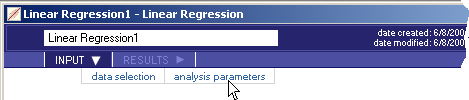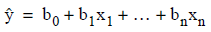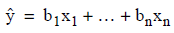# 5.4.5 - Linear Regression - INPUT - Analysis Parameters - Teradata Warehouse Miner

## Teradata Warehouse Miner User Guide - Volume 3Analytic Functions

Product
Release Number
5.4.5
Published
February 2018
Language
English (United States)
Last Update
2018-05-04
dita:mapPath
yuy1504291362546.ditamap
dita:ditavalPath
ft:empty
1. On the Linear Regression dialog box, click INPUT.
2. Click analysis parameters.
Linear Regression > Input > Analysis Parameters3. On this screen, select:
• Regression Options
• Include Constant — This option specifies that the linear regression model includes a constant term. With a constant, the linear equation can be thought of as:Without a constant, the equation changes to:• Stepwise Options — The Linear Regression analysis can use the stepwise technique to automatically determine a variable’s importance (or lack there of) to a particular model. If selected, the algorithm is performed repeatedly with various combinations of independent variable columns to attempt to arrive at a final “best” model. The stepwise options are:
• Step Direction — (Selecting “None” turns off the Stepwise option).
• Forward Only — Option to add qualifying independent variables one at a time.
• Forward — Option for independent variables being added one at a time to an empty model, possibly removing a variable after a variable is added.
• Backward Only — Option to remove independent variables one at a time.
• Backward — Option for variables being removed from an initial model containing all of the independent variables, possibly adding a variable after a variable is removed.
• Step Method
• F Statistic — Option to choose the partial F test statistic (F statistic) as the basis for adding or removing model variables.
• P-value — Option to choose the probability associated with the T-statistic (P-value) as the basis for adding or removing model variables.
• Criterion to Enter
• Criterion to Remove — If the step method is to use the F statistic, then an independent variable is only added to the model if the F statistic is greater than the criterion to enter and removed if it is less than the criterion to remove. When the F statistic is used, the default for each is 3.84.

If the step method is to use the P-value, then an independent variable is added to the model if the P-value is less than the criterion to enter and removed if it is greater than the criterion to remove. When the P-value is used, the default for each is 0.05.

The default F statistic criteria of 3.84 corresponds to a P-value of 0.05. These default values are provided with the assumption that the input variables are somewhat correlated. If this is not the case, a lower F statistic or higher P-value criteria can be used. Also, a higher F statistic or lower P value can be specified if more stringent criteria are desired for including variables in a model.

• Report Options — Statistical diagnostics can be taken on each variable during the execution of the Linear Regression Analysis. These diagnostics include:
• Variable Statistics — This report gives the mean value and standard deviation of each variable in the model based on the SSCP matrix provided as input.
• Near Dependency — This report lists collinear variables or near dependencies in the data based on the SSCP matrix provided as input.
• Condition Index Threshold — Entries in the Near Dependency report are triggered by two conditions occurring simultaneously. The one that involves this parameter is the occurrence of a large condition index value associated with a specially constructed principal factor. If a factor has a condition index greater than this parameter’s value, it is a candidate for the Near Dependency report. A default value of 30 is used as a rule of thumb.
• Variance Proportion Threshold — Entries in the Near Dependency report are triggered by two conditions occurring simultaneously. The one that involves this parameter is when two or more variables have a variance proportion greater than this threshold value for a factor with a high condition index. Another way of saying this is that a ‘suspect’ factor accounts for a high proportion of the variance of two or more variables. This parameter defines what a high proportion of variance is. A default value of 0.5 is used as a rule of thumb.
• Detailed Collinearity Diagnostics — This report provides the details behind the Near Dependency report, consisting of the “Eigenvalues of Unit Scaled X’X”, “Condition Indices” and “Variance Proportions” tables.